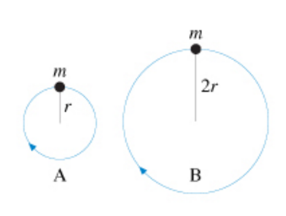# Problem: The figure (figure 1) shows two balls of equal mass moving in vertical circles Is the tension in string A greater than less than, or equal to the tension in string B if the balls travel over the top of the circle with equal angular velocity?a. The tension in string A is greater than the tension in string Bb. The tension in string A is less than the tension in string Bc. The tension in string A is equal to the tension in string B

###### FREE Expert Solution

Angular frequency:

$\overline{){\mathbf{\omega }}{\mathbf{=}}\frac{\mathbf{v}}{\mathbf{r}}}$

Centripetal force:

m and ω are the same for both.

84% (401 ratings)###### Problem Details

The figure (figure 1) shows two balls of equal mass moving in vertical circlesIs the tension in string A greater than less than, or equal to the tension in string B if the balls travel over the top of the circle with equal angular velocity?

a. The tension in string A is greater than the tension in string B

b. The tension in string A is less than the tension in string B

c. The tension in string A is equal to the tension in string B

Frequently Asked Questions

What scientific concept do you need to know in order to solve this problem?

Our tutors have indicated that to solve this problem you will need to apply the Rotational Velocity & Acceleration concept. You can view video lessons to learn Rotational Velocity & Acceleration. Or if you need more Rotational Velocity & Acceleration practice, you can also practice Rotational Velocity & Acceleration practice problems.

What professor is this problem relevant for?

Based on our data, we think this problem is relevant for Professor Spyrou's class at MSU.